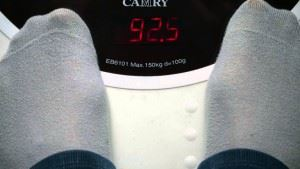# Applications Of Decimal In Daily Life

We use decimals every day while dealing with money, weight, length etc. Decimal numbers are used in situations where more precision is required than the whole numbers can provide. For example, when we calculate our weight on the weighing machine, we do not always find the weight equal to a whole number on the scale. In order to know our exact weight, we must understand what the decimal value on the scale means. This section deals with the concept of decimals in three important fields of our daily life.• Use of decimal to represent money

Dealing with decimal numbers is inevitable when dealing with money. In many situations when we have to convert paisa into rupee. Suppose we go to a local shop to buy 500 gm of turmeric, where, one kg of turmeric costs Rs 51. So, how much money should we hand over to the shopkeeper? We divide Rs 51 by 2 which are equal to 25.5. In order to hand over the exact amount, we must understand what 25.5 means in terms of rupees. Let us learn this with the help of a simple example.

Re 1 = 100 paisa

Example 1: Converting 165 paisa to Re.

As we know, 1 paisa = 1/100 Re

So, 165 paisa = Re 165*1/100 = Re 165/100 = Rs 1.65, which is Re 1 and 65 paisa

Example 2: Converting 450 paisa to Re.

As we know, 1 paisa = Re 1/100

So, 450 paisa = 450*1/100 = Rs 450/100 = Rs 4.50 = Rs 4 and 50 paisa

Example 3: Converting Rs 35 and 70 paisa to decimal.

As we know, 1 paisa = Re 1/100

So, 70 paisa = 70*1/100

Rs (35 + 70/100) = Rs 35.70

• Use of decimal to represent the length

While measuring the length of an item, it is not necessary that the length of an object is a multiple of the given graduation. For example, while measuring the length of a table with a metre scale, the length may not be a whole number, it may lie between two graduations on the metre scale. In such situations, the decimal numbers are used.

From Conversion of units, we know

1 km = 100 m

1 m = 100 cm

1 cm = 10 mm

Now, let the length of the table top be 2 m and 75 cm, and then it can be represented as (2+ 75/100) m.

Example 4: Converting 276 cm into meters.

As we know, 100 cm = 1 m

So, 1 cm = 1/100 m

276 cm = 276 * 1/100 m = 276/100 m = 2.76 m

Example 5: Converting 5km and 75 m into decimal.

As we know, 1 km = 1000 m

So, 1 m = 1/1000 km

5 km + 75m = 5+ (75*1/1000) km = 5.075 km

Example 6: Converting 80 mm to km

As we know 1 km = 1000 m and 1 m = 100 cm

So 1 km = 1000 * 100 cm

1 cm = 1/10000km

80 cm = 80*1/100000 km

80 cm = 0.00080 km

• Use of decimal to represent weight

We use decimal numbers while dealing with weight. For example, when we are buying a watermelon, it cannot always weigh in whole numbers, it can be less than 2 kg but more than 1kg. In such situations, the shopkeeper has to calculate how much to charge for a watermelon, based on its weight. As we know,

1 kg = 1000 gm

1 gm = 1000 mg

Now suppose it is 1kg and 750 gm. Then, he will charge as per the price of 1kg + (750/1000) kg of the watermelon. We will learn more about the conversion of weight into decimal in the following examples:

Example 7: Converting 250 gm to kg

As we know, 1000 gm = 1 kg

So, 1 gm = 1/1000 kg

250 gm = 250 * 1/1000 kg = 250/1000 kg = 0.250 kg

Example 8: Representing 3kgs and 767 gm in decimal.

As we know, 1gm = 1/1000 kg

767gm = 767/1000 kg

So, 3kg + 767/1000 kg = 3.767 kg

To know more about decimal representation, visit BYJU’S.